# The HPLOGISTIC Procedure

### OUTPUT Statement

• OUTPUT <OUT=SAS-data-set><COPYVARS=(variables)><keyword <=name>>…<keyword <=name>> </ options>;

The OUTPUT statement creates a data set that contains observationwise statistics that PROC HPLOGISTIC computes after fitting the model. The variables in the input data set are not included in the output data set, in order to avoid data duplication for large data sets; however, variables that you specify in the ID statement or COPYVAR= option are included.

If the input data are in distributed form, where access of data in a particular order cannot be guaranteed, the HPLOGISTIC procedure copies the distribution or partition key to the output data set so that its contents can be joined with the input data.

The output statistics are computed based on the final parameter estimates. If the optimization does not converge, then the output data set is not created.

When there are more than two response levels, values are computed only for variables that are named by the XBETA, POST, and PRED keywords; the other variables have missing values. These statistics are computed for every response category, and the automatic variable _LEVEL_ identifies the response category upon which the computed values are based. That is, every observation generates several rows in the output data set. If you also specify the OBSCAT option, then the observationwise statistics are computed only for the observed response category, as indicated by the value of the _LEVEL_ variable.

For observations in which only the response variable is missing, values of the XBETA, POST, and PRED statistics are computed even though these observations do not affect the model fit. This enables, for instance, predicted probabilities to be computed for new observations.

You can specify the following syntax elements in the OUTPUT statement before the slash (/).

OUT=SAS-data-set
DATA=SAS-data-set

specifies the name of the output data set. If the OUT= (or DATA=) option is omitted, the procedure uses the DATAn convention to name the output data set.

COPYVAR=variable
COPYVARS=(variables)

transfers one or more variables from the input data set to the output data set. Variables named in an ID statement are also copied from the input data set to the output data set.

keyword <=name>

specifies a statistic to include in the output data set and optionally names the variable name. If you do not provide a name, the HPLOGISTIC procedure assigns a default name based on the type of statistic requested.

The following are valid keywords for adding statistics to the OUTPUT data set:

LINP | XBETA

requests the linear predictor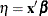. The default name is Xbeta.

PEARSON | PEARS | RESCHI

requests the Pearson residual,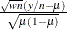, where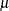is the estimate of the predicted event probability, w is the weight of the observation, and n is the number of binomial trials (n=1 for binary observations). The default name is Pearson. This statistic is not computed for multinomial models.

POSTERIOR | POST

requests a numeric variable that contains the posterior predicted probability of each observation that is used in fitting the model. The default name is _POST_. If you do not specify the PRIOR option in the MODEL statement, then this value is the same as the predicted probability.

PREDICTED | PRED | P

requests predicted values (predicted probabilities of events) for the response variable. The default name is Pred.

RESIDUAL | RESID | R

requests the raw residual,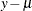, whereis the estimate of the predicted event probability. The default name is Residual. This statistic is not computed for multinomial models.

ROLE

requests a numeric variable that indicates the role played by each observation in fitting the model. The default name is _ROLE_. Table 10.5 shows how this variable is interpreted for each observation.

Table 10.5: Role Interpretation

Value

Observation Role

0

Not used

1

Training

2

Validation

3

Testing

If you do not partition the input data by specifying a PARTITION statement, then the role variable value is 1 for observations that are used in fitting the model and 0 for observations that have at least one missing or invalid value for the response, regressors, frequency or weight variables.

You can specify the following options in the OUTPUT statement after the slash (/):

ALLSTATS

adds all available statistics to the output data set.

OBSCAT

requests (for multinomial models) that observationwise statistics be produced for the response level only. If you do not specify the OBSCAT option and the response variable has J levels, then the following outputs are created: for cumulative link models,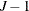records are output for every observation in the input data that corresponds to thelower-ordered response categories; for generalized logit models, J records are output that correspond to all J response categories.